# How to find Top or Bottom N values in Excel?

• Last Updated : 24 Feb, 2022

Functions help find top or bottom N values in excel very easily. The functions used to find Nth largest and Nth smallest number are = LARGE(array, k) and = SMALL(array, k) respectively.

### Cell Reference

Before understanding the LARGE and SMALL functions. We first need to have a fine knowledge of cell references. Cell reference is of two types:

• Relative Reference

Relative reference is the reference that changes with cells.

1. If we try dragging the same formula down across a column then numbers will increase.
2. If we try dragging the same formula side across a row, then alphabets will increase.

Here is an example for better understanding: Consider a data set of Students with their Physics and Math marks. Calculate the total marks.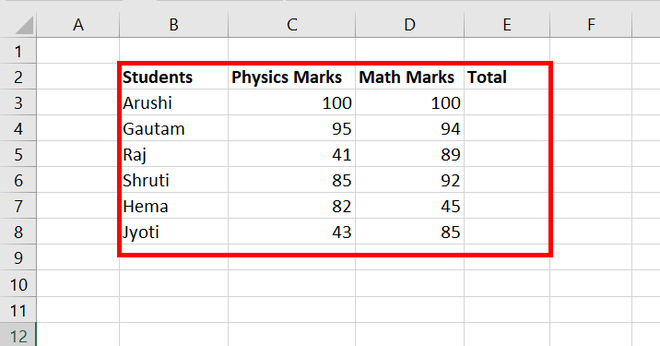Steps for finding total

Step 1: Write a formula for sum i.e. = SUM(value1, value2) and press Enter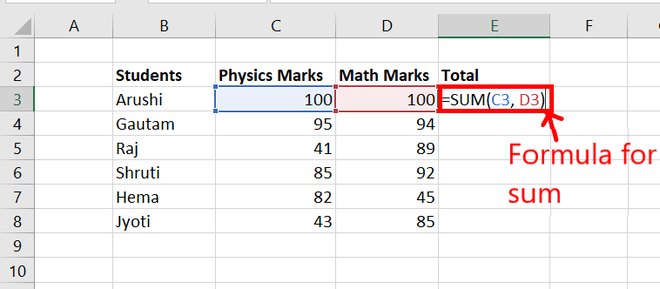Step 2: Try dragging the same formula downward and hence we get the sum of all students.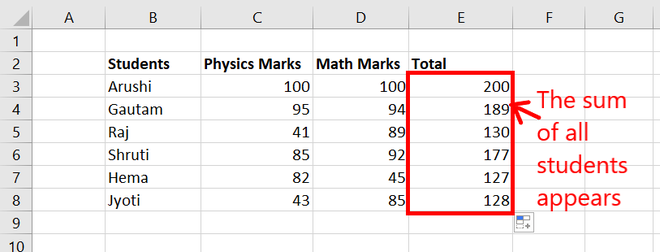Step 3: Go to Formula Tab and click on Show Formulas. Now, all the formulas of the given worksheet will appear.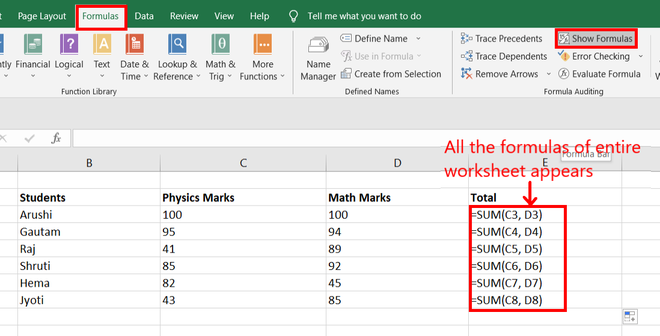Step 4: We find in range E3:E9 that the values inside the functions are increasing as we go downward in a row. For Example: in cell E3, the formula is =SUM(C3, D3) and in cell E4, the formula is =SUM(C4, D4).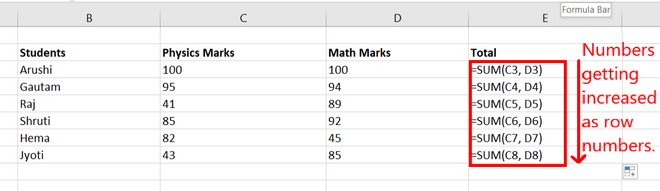• Absolute Reference

The absolute reference remains static even if the rows or columns are changing. There can be two ways to make a selected cell with absolute reference:

1. Use \$ symbol: Write \$ after each and every character of the selected cells.
2. Use F4: Press Fn + F4 on your keyboard to make absolute reference to a selected cell.

Consider the same data set as above. Try using the same formula for total but with absolute reference.

Steps of finding total with absolute reference

Step 1: Considering the same data set and write the function with absolute reference.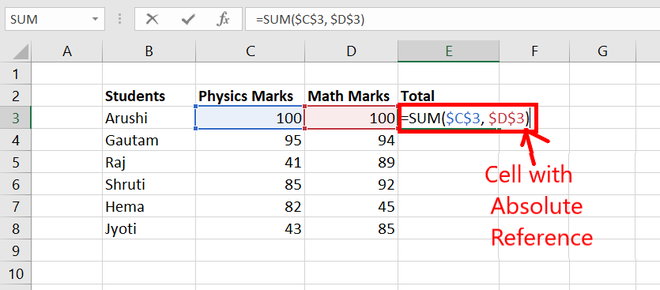Step 2: Now, go to Formulas Tab and click on Show Formulas. All formulas of the given worksheet appear.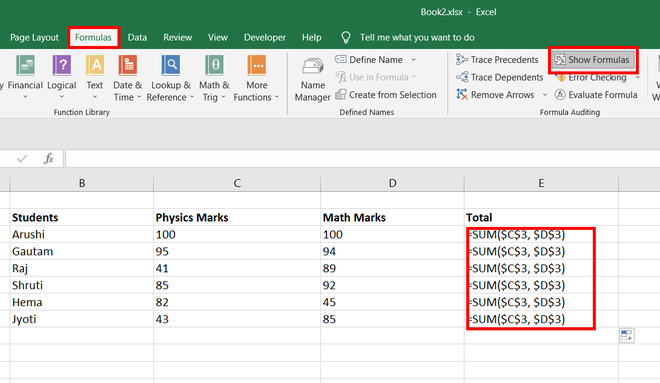Step 3: We observe that while going downward in a row the selected cells remain the same.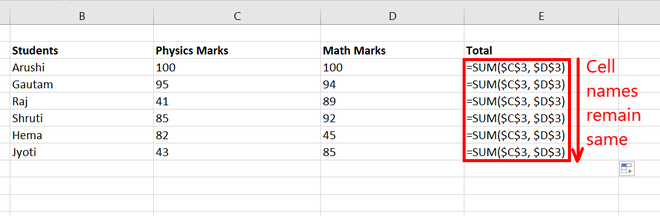### Top N values

Given a data set of Students and their Marks. Try finding the highest 3 marks scored by students.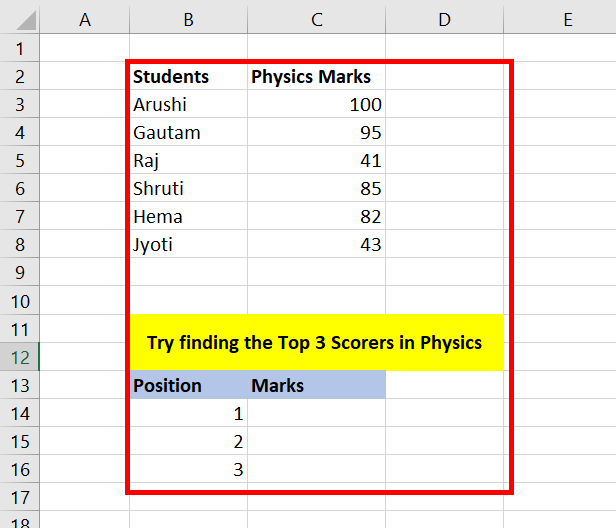Steps for top N values

Step 1: Use = LARGE(Array, k) function to have kth largest number in an array. Press Enter

• Array: It is the first argument of the LARGE function. We need to provide an absolute reference for this array.
• k: It is the second argument of the LARGE function. It specifies which largest number you want. We will provide a relative reference to this number.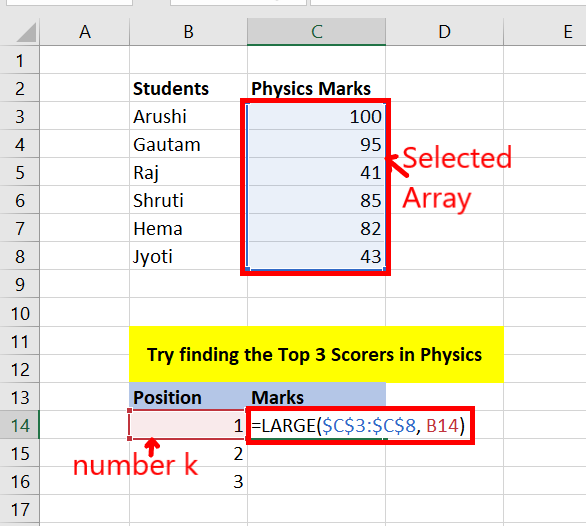Step 2: You will get the kth largest number from the array. Now, drag down till the N numbers you want. For example: drag down to 3 cells for the given data set.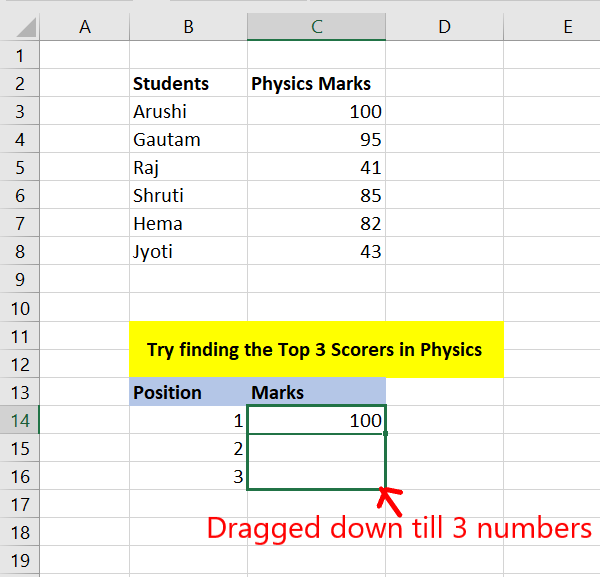Step 3: You have obtained the highest 3 marks scored by students.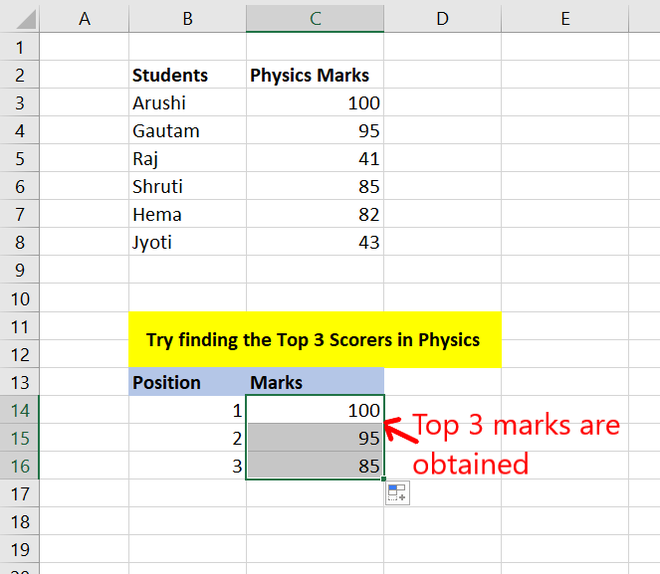### Bottom N values

Consider the same data set of Students and their Marks. Try finding the lowest 3 marks obtained by students.

Steps for bottom N values

Step 1: Use = SMALL(Array, k) function to have kth smallest number in an array. For example, if the value of k is 1, then the function will return the smallest number in the array. If the value of k is 2, then the function will return the second smallest number in the array. Press Enter

• Array: It is the first argument of the SMALL function. We need to provide an absolute reference for this array.
• k:  It is the second argument of the SMALL function. It specifies which smallest number you want. We will provide a relative reference to this number.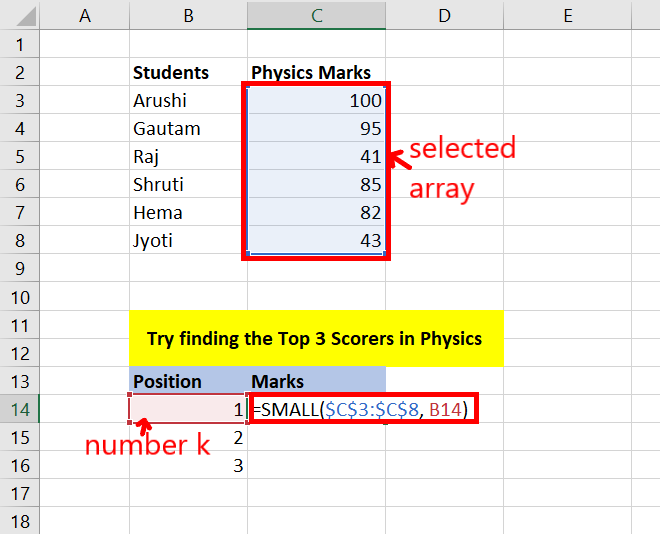Step 2: You will get the kth smallest number from that array. Now, drag down till the N numbers you want. For example: drag down to 3 cells for the given data set.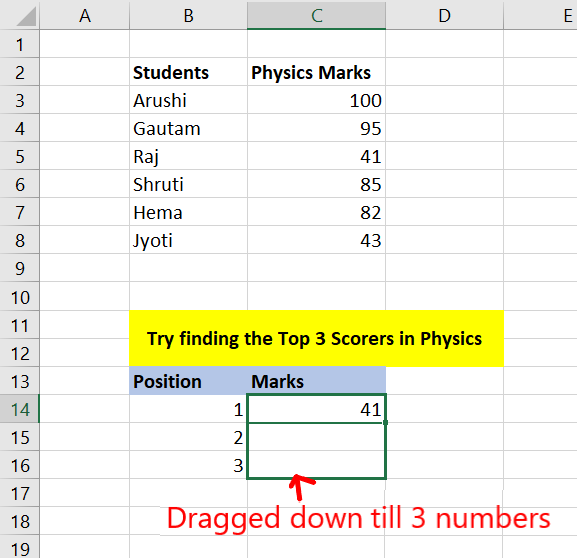Step 3: You have obtained the lowest 3 marks scored by students.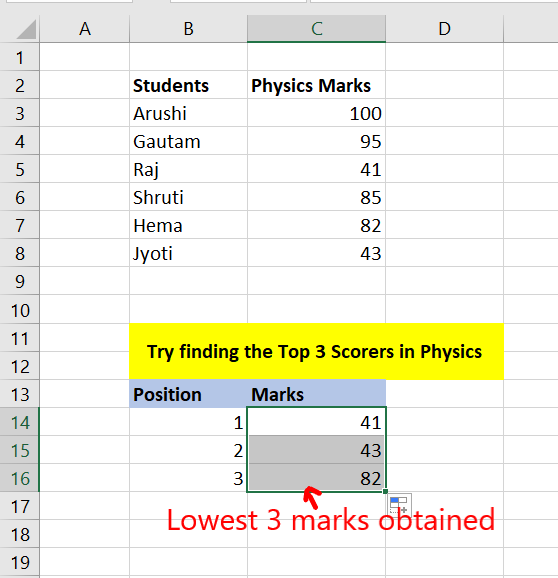My Personal Notes arrow_drop_up
Recommended Articles
Page :